# VideoDreamer: Customized Multi-Subject Text-to-Video Generation with Disen-Mix Finetuning

Hong Chen, Xin Wang, Guanning Zeng, Yipeng Zhang, Yuwei Zhou, Feilin Han, Wenwu Zhu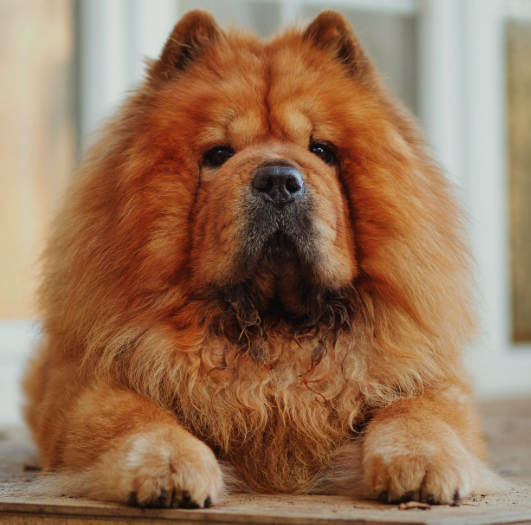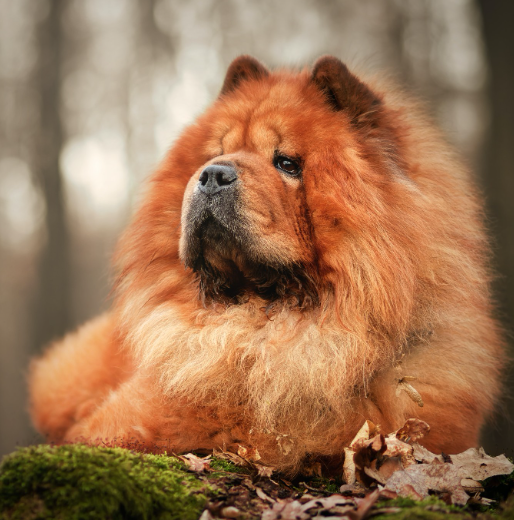$Subject1:\ A\ S_1^*\ dog$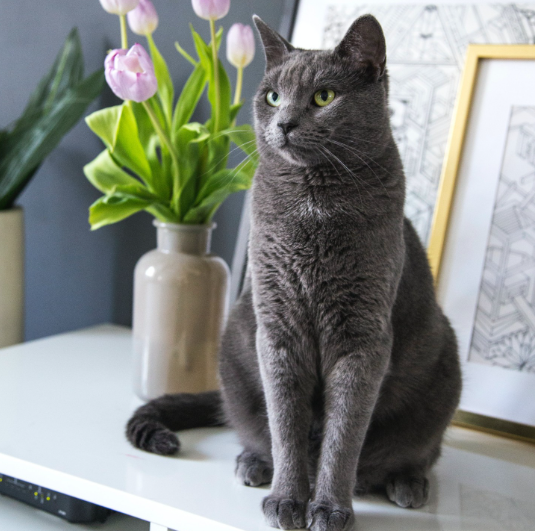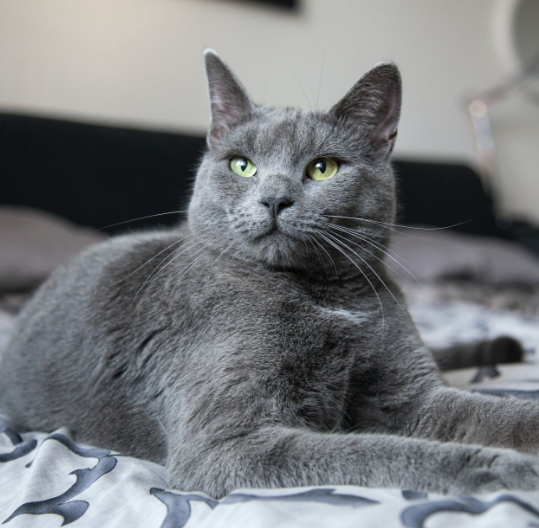$Subject2:\ A\ S_2^*\ cat$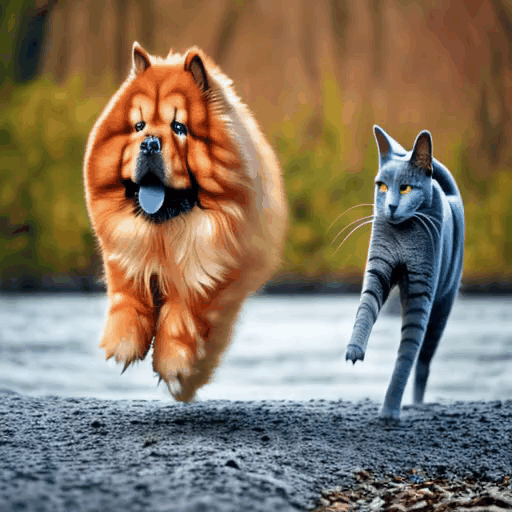$A\ S_1^*\ dog\ and\ a\ S_2^*\$ $cat\ are\ jumping\$ $over\ a\ river.$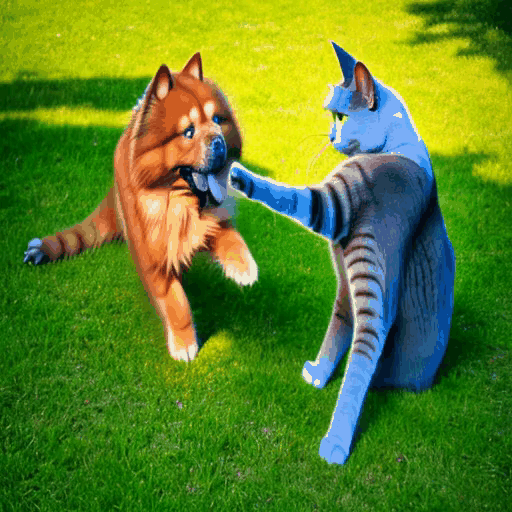$A\ S_1^*\ dog\ and\ a\ S_2^*\ cat\$ $are\ dancing\ on\ the$ $grass,\ waving\ feet.$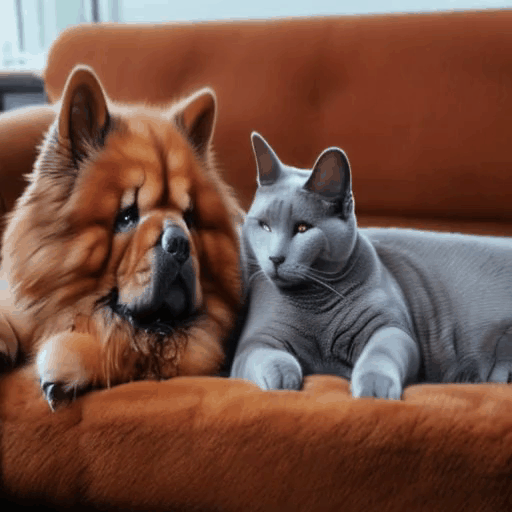$A\ S_1^*\ dog\ and\ a\ S_2^*\$ $cat\ are\ lying\ on\ the\$ $sofa\ together$$Subject1:\ A\ S_1^*\ girl$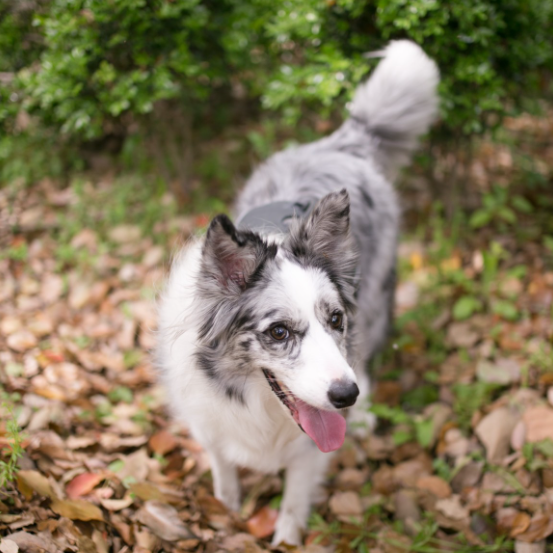$Subject2:\ A\ S_2^*\ dog$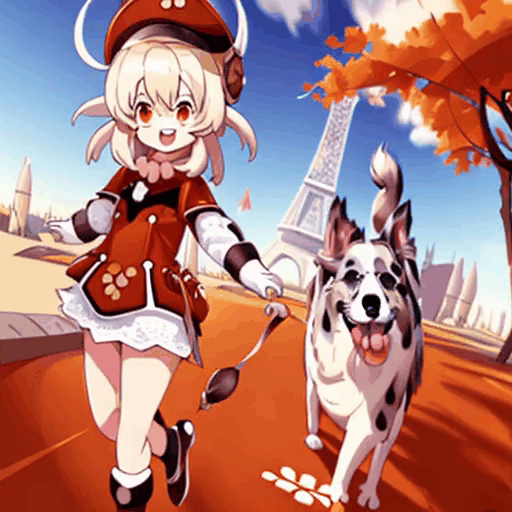$A\ S_1^*\ girl\ and\ a\ S_2^*\$ $dog\ are\ walking\ under$ $the\ Eiffel\ tower.$$A\ S_1^*\ girl\ and\ a\ S_2^*\$ $dog\ are\ skiing\ down$ $the\ hill\ on\ board.$$A\ S_1^*\ girl\ and\ a\ S_2^*\$ $dog\ are\ surfing\$ $in\ the\ ocean.$
Customized multi-subject text-to-video generation results by VideoDreamer. Given multiple subjects and few images for each subject, our VideoDreamer can generate videos that contain the given subjects and simultaneously conform to new text prompts.

## Abstract

Customized text-to-video generation aims to generate text-guided videos with customized user-given subjects, which has gained increasing attention recently. However, existing works are primarily limited to generating videos for a single subject, leaving the more challenging problem of customized multi-subject text-to-video generation largely unexplored. In this paper, we fill this gap and propose a novel VideoDreamer framework. VideoDreamer can generate temporally consistent text-guided videos that faithfully preserve the visual features of the given multiple subjects. Specifically, VideoDreamer leverages the pretrained Stable Diffusion with latent-code motion dynamics and temporal cross-frame attention as the base video generator. The video generator is further customized for the given multiple subjects by the proposed Disen-Mix Finetuning and Human-in-the-Loop Re-finetuning strategy, which can tackle the attribute binding problem of multi-subject generation. We also introduce MultiStudioBench, a benchmark for evaluating customized multi-subject text-to-video generation models. Extensive experiments demonstrate the remarkable ability of VideoDreamer to generate videos with new content such as new events and backgrounds, tailored to the customized multiple subjects.

## Framework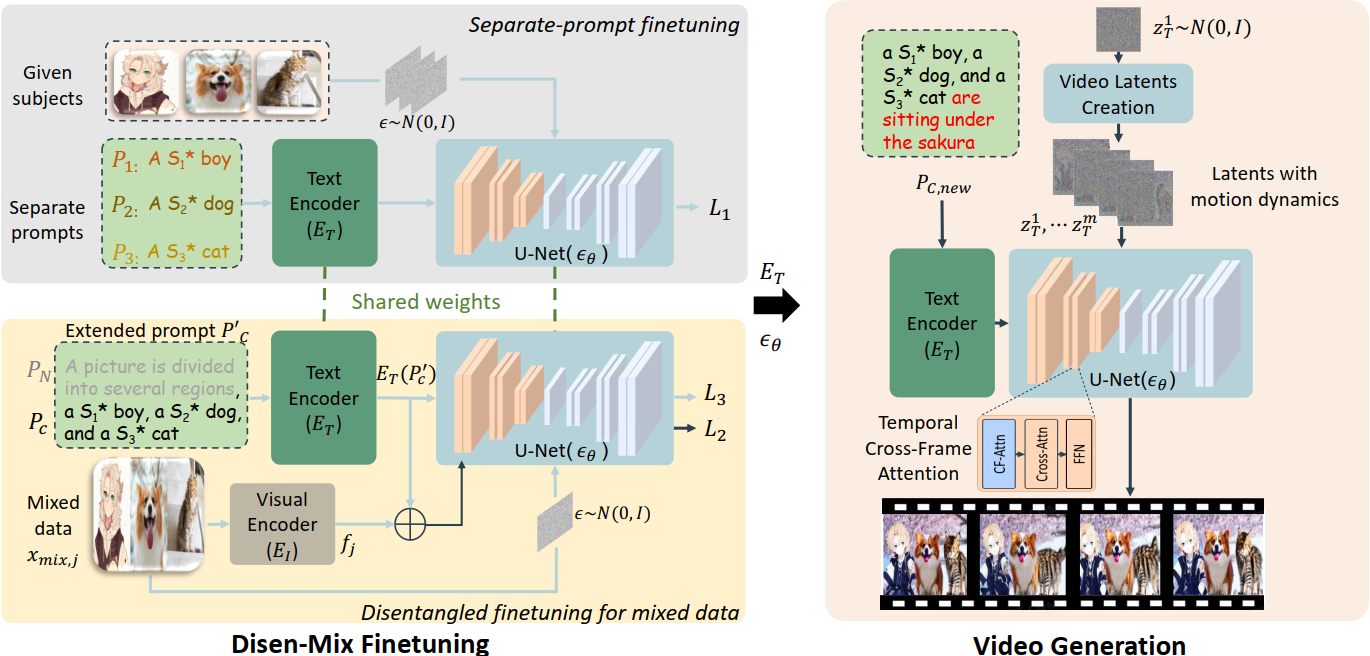VideoDreamer leverages the Stable Diffusion (Text Encoder and U-Net) as the video generator as shown in the right figure, where the motion dynamics and temporal cross-frame attention are used to maintain temporal consistency among the generated frames. To achieve the multi-subject customization, in the left figure, we design the separate-prompt finetuning for customizing the model with each subject, while the disentangled finetuning for mixed data is designed to avoid the attribute binding problem when generating multiple subjects in the same frame. The extended prompt and the visual encoder are designed to avoid the artificial stitches brought by the mixed data.

## VideoDreamer Generated Examples

VideoDreamer can customize different multiple subjects, and generate videos with different events, which could serve as a useful tool for customized multi-subject text-to-video generation.Given subjects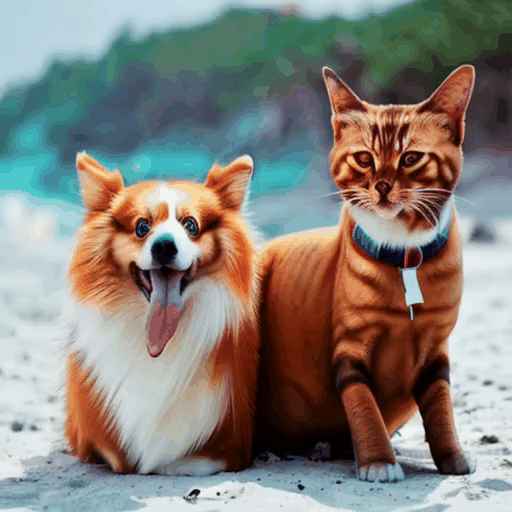$A\ S_1^*\ dog\ and\ a\ S_2^*\ cat\$ $are\ sitting\ on\ the\ beach\$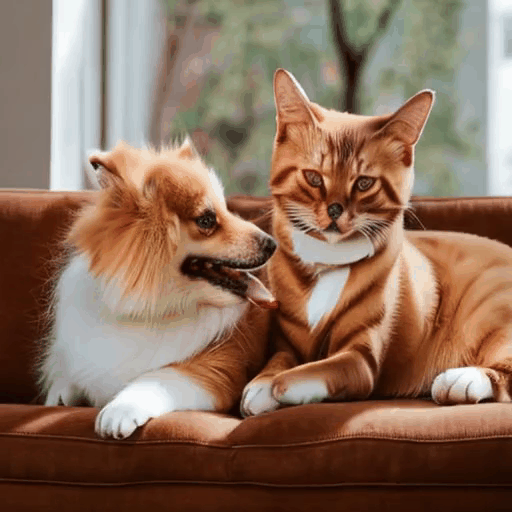$A\ S_1^*\ dog\ and\ a\ S_2^*\ cat\$ $are\ lying\ on\ the\ sofa$Given subjects$A\ S_1^*\ bear\ and\ a\ S_2^*\ bear\$ $are\ dancing\ in\ the\ forest$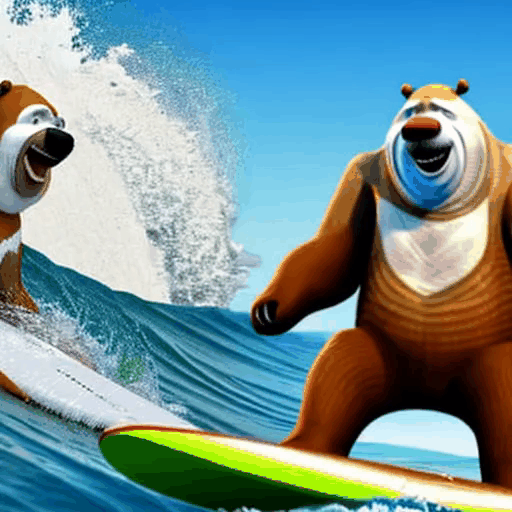$A\ S_1^*\ bear\ and\ a\ S_2^*\ bear\ are\$ $surfing\ over\ the\ waves$Given subjects$A\ S_1^*\ boy\ and\ a\ S_2^*\ cat\ are\$ $playing\ the\ guitar$$A\ S_1^*\ boy\ and\ a\ S_2^*\ cat$ $are\ playing\ chess$Given subjects$A\ S_1^*\ girl\ and\ a\ S_2^*\ girl\$ $are\ sitting\ on\ the\ sofa\$$A\ S_1^*\ girl\ and\ a\ S_2^*\ girl\ are\$ $driving\ a\ car$Given subjects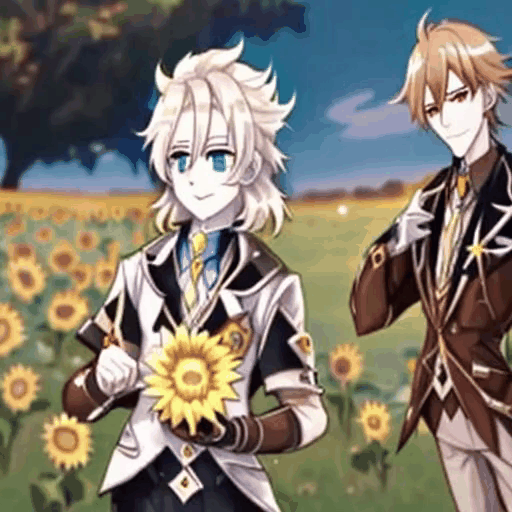$A\ S_1^*\ boy\ and\ a\ S_2^*\ boy\ are$ $\ walking\ on\ the\ sunflower\ grass$$A\ S_1^*\ boy\, and\ a\ S_2^*\ boy\ are$ $lying\ on\ the\ sofa$Given subjects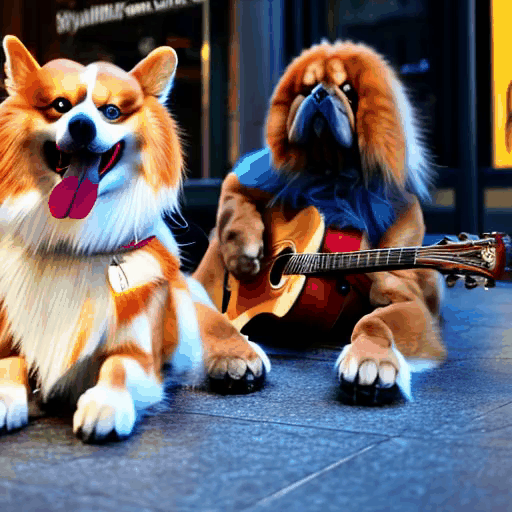$A\ S_1^*\ dog\ and\ a\ S_2^*\ dog\$ $are\ playing\ the\ guitar$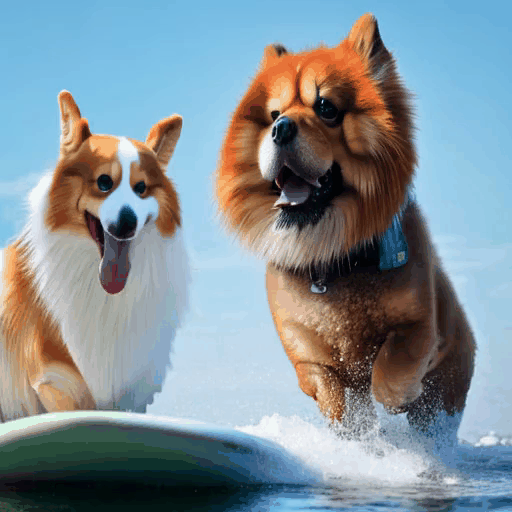$A\ S_1^*\ dog\ and\ a\ S_2^*\ dog\ are\$ $surfing\ in\ the\ ocean$Given subjects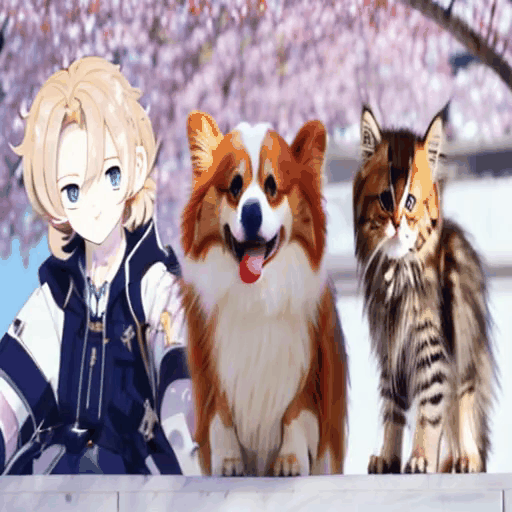$A\ S_1^*\ boy,\ a\ S_2^*\ dog,\ and$ $a\ S_3^*\ cat\ are\ sitting$ $under\ the\ sakura$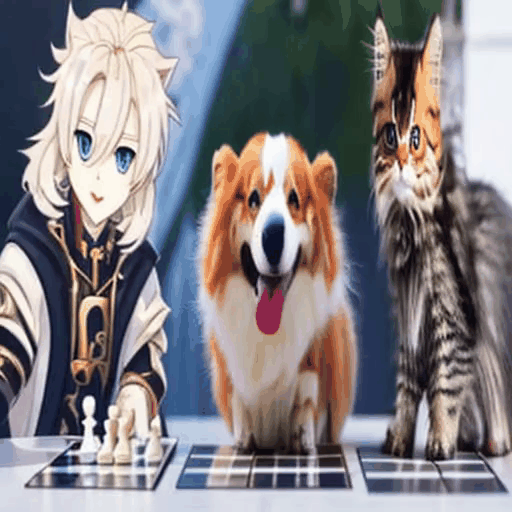$A\ S_1^*\ boy,\ a\ S_2^*\ dog,$ $and\ a\ S_3^*\ cat\ are$ $playing\ chess$Given subjects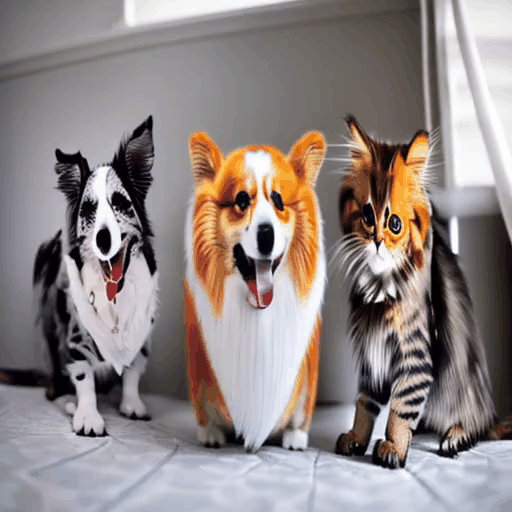$A\ S_1^*\ dog,\ a\ S_2^*\ dog,\ and$ $a\ S_3^*\ cat\ are\ sitting\ in$ $the\ kitchen$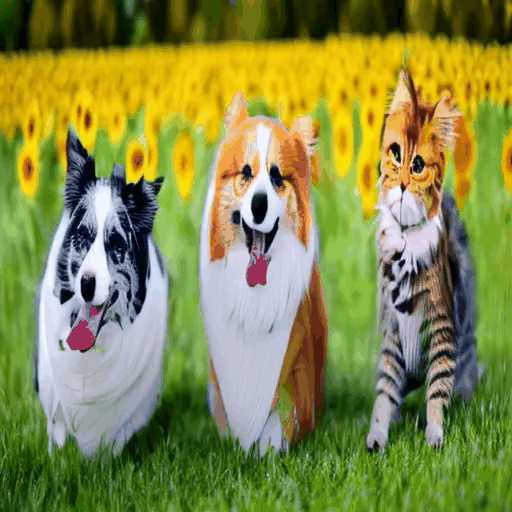$A\ S_1^*\ dog,\ a\ S_2^*\ dog,\ and$ $a\ S_3^*\ cat\ are\ running\ on$ $the\ grass\ with\ sunflowers$

## Qualitative Comparison with Baselines

Compared with the baselines, VideoDreamer can better preserve the identity of each subject than the baselines. Additionally, VideoDreamer will introduce no additional artifacts.

Baselines
VideoDreamer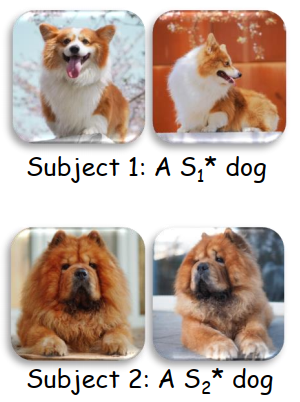Given subjects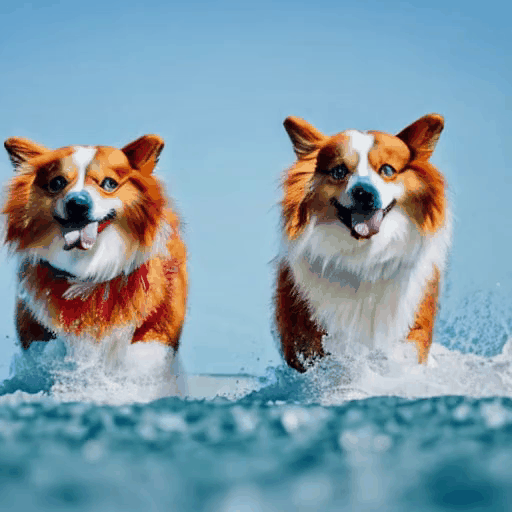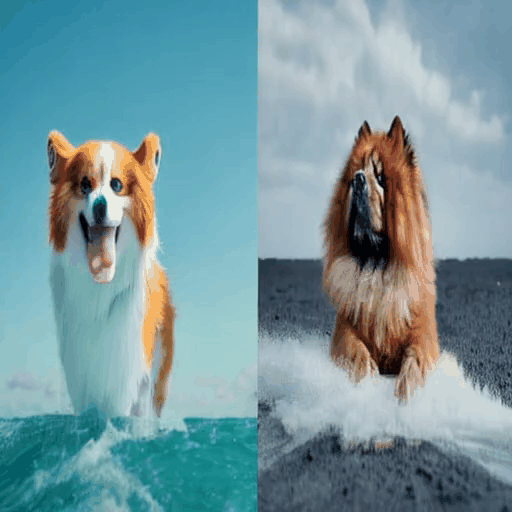$A\ S_1^*\ dog\ and\ a\ S_2^*\ dog\ are\ surfing\ in\ the\ sea\$Given subjects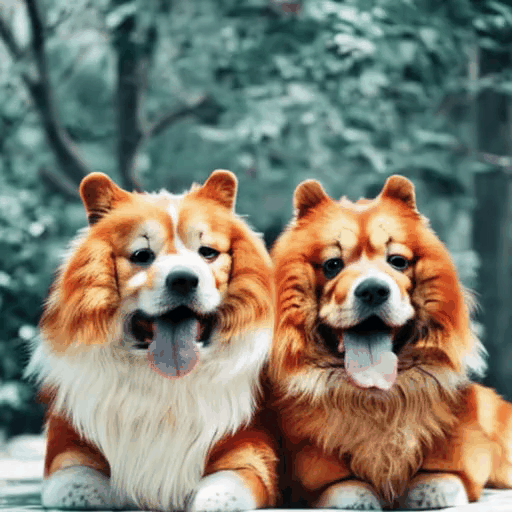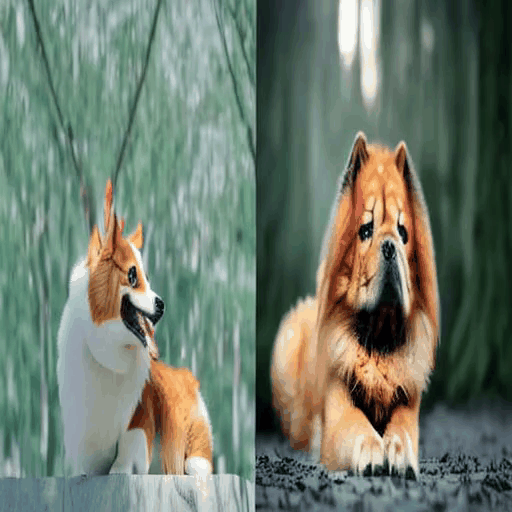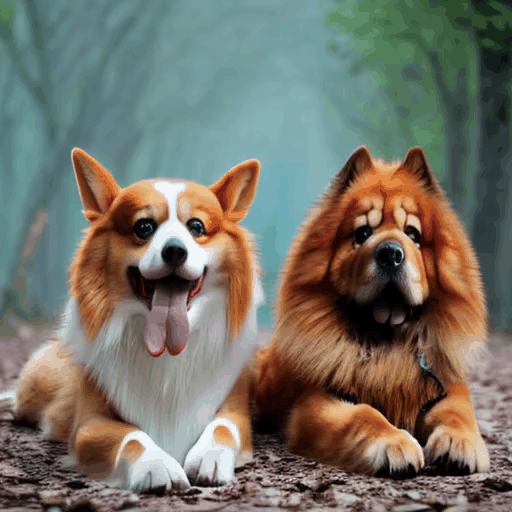$A\ S_1^*\ dog\ and\ a\ S_2^*\ dog\ are\ sitting\ in\ the\ forest\$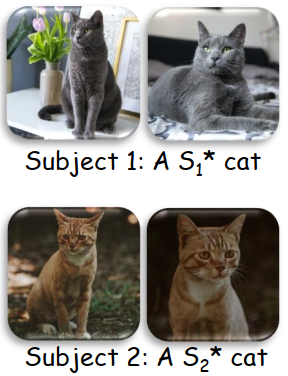Given subjects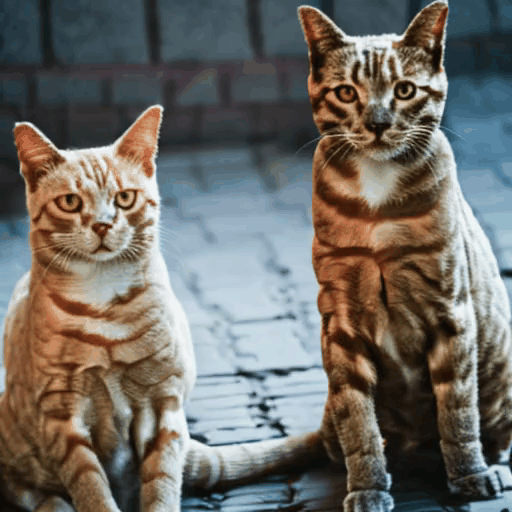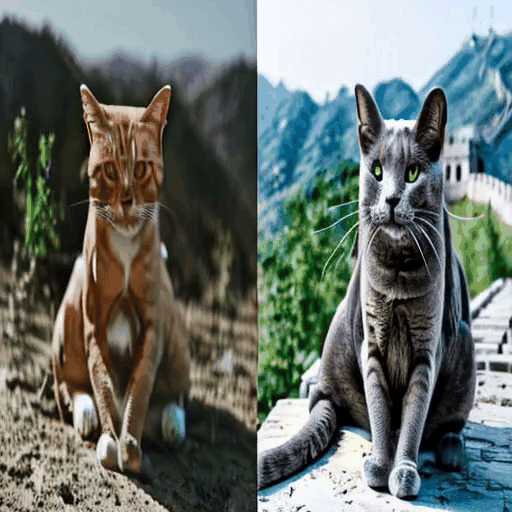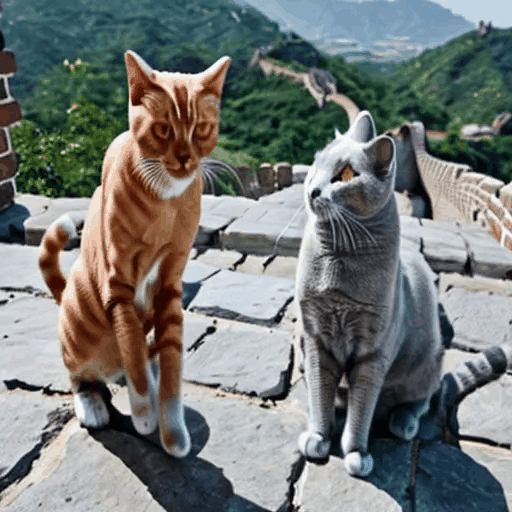$A\ S_1^*\ dog\ and\ a\ S_2^*\ cat\ are\ walking\ on\ the\ Great\ Wall$Given subjects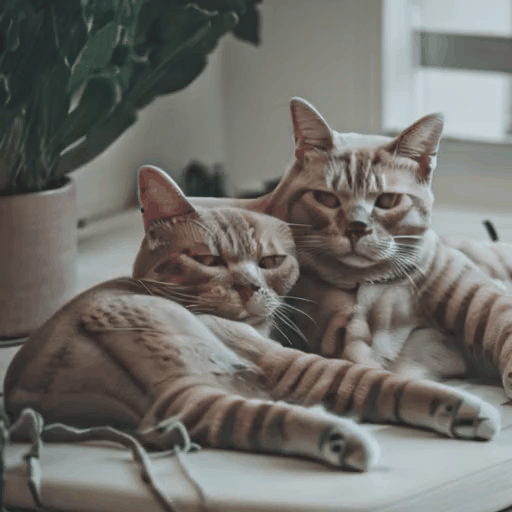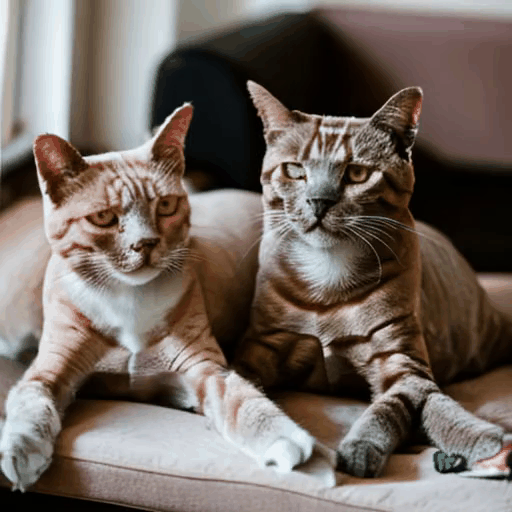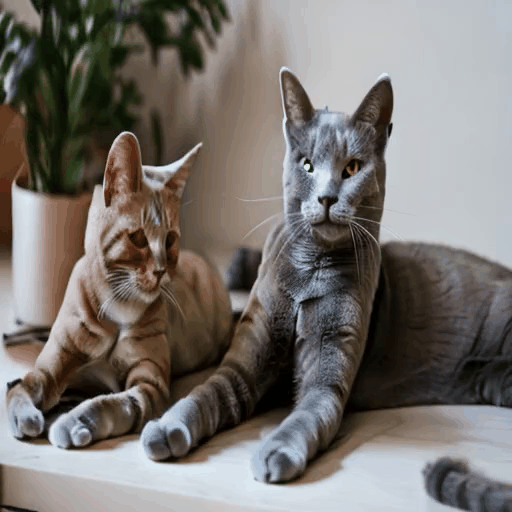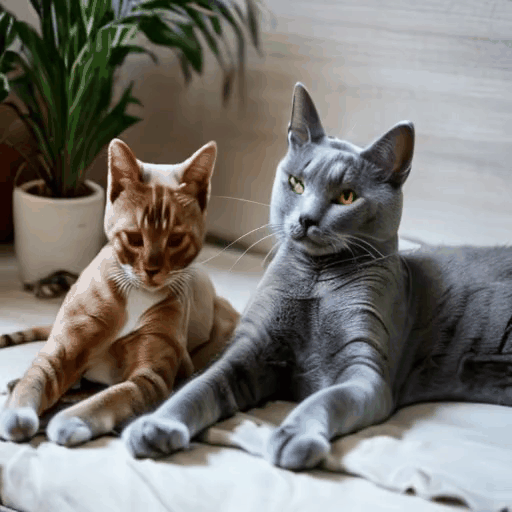$A\ S_1^*\ dog\ and\ a\ S_2^*\ cat\ are\ lying\ in\ the\ kitchen$

## BibTeX


@misc{chen2023videodreamer,
title={VideoDreamer: Customized Multi-Subject Text-to-Video Generation with Disen-Mix Finetuning},
author={Hong Chen and Xin Wang and Guanning Zeng and Yipeng Zhang and Yuwei Zhou and Feilin Han and Wenwu Zhu},
year={2023},
eprint={2311.00990},
archivePrefix={arXiv},
primaryClass={cs.CV}
}